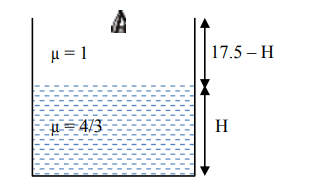# A glass tumbler having inner depth of 17.5cm is kept on a table.`
Question:

A glass tumbler having inner depth of $17.5 \mathrm{~cm}$ is kept on a table. A student starts pouring water $(\mu=4 / 3)$ into it while looking at the surface of water from the above. When he feels that the tumbler is half filled, he stops pouring water. Up to what height, the tumbler is actually filled ?

1. 11.7 cm

2. 10 cm

3. 7.5 cm

4. 8.75 cm

Correct Option: , 2

Solution:Height of water observed by observer

$=\frac{H}{\mu_{w}}=\frac{H}{(4 / 3)}=\frac{3 H}{4}$

Height of air observed by observer = 17.5 – H According to question, both height observed by observer is same.

$\frac{3 \mathrm{H}}{4}=17.5-\mathrm{H}$

$\Rightarrow \mathrm{H}=10 \mathrm{~cm}$